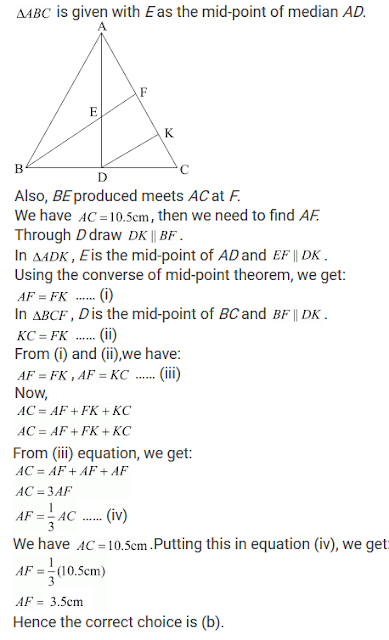#### Chapter 14 Quadrilaterals R.D. Sharma Solutions for Class 9th MCQ's

Multiple Choice Questions

Mark the correct alternative in each of the following:

1. The opposite sides of a quadrilateral have

(a) no common point
(b) one common point
(c) two common points
(d) infinitely many common points

Solution2. The consecutive sides of a quadrilateral have
(a) no common point
(b) one common point
(c) two common points
(d) infinitely many common points

Solution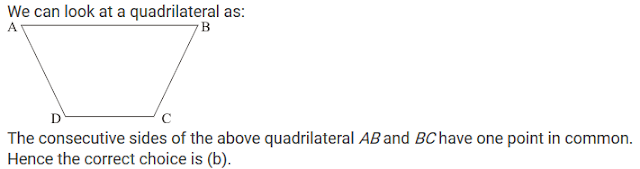3. PQRS is a quadrilateral, PR and QS intersect each other at O. In which of the following cases, PQRS is a parallelogram?
(a) ∠P = 100°, ∠Q = 80°, ∠R = 95°
(b) ∠P =85°, ∠Q = 85°, ∠R = 95°
(c) PQ = 7 cm, QR = 7 cm, RS = 8 cm, SP = 8 cm
(d) OP = 6.5 cm, OQ = 6.5 cm, OR = 5.2 cm, OS = 5.2 cm

Solution

Let us analyze each case one by one.
We have a quadrilateral named PQRS, with diagonals PR and QS intersecting at O.
(a) ∠P=100°, ∠Q = 80°, ∠R=100°
By angle sum property of a quadrilateral, we get:
∠P + ∠Q + ∠R + ∠S = 360°
⇒ 100° +80° +100° + ∠S = 360°
⇒ 280° + ∠S = 360°
⇒ ∠S - 80°
Clearly, ∠P = ∠R
And, ∠Q = ∠S
Thus we have PQRS a quadrilateral with opposite angles are equal.
Therefore, PORS is a parallelogram.

(b) ∠P = 85°, ∠Q=85°, ∠R=95°
By angle sum property of a quadrilateral, we get:
∠P + ∠Q + ∠R + ∠S = 360°
⇒ 85° +85° +95° + ∠S = 360°
⇒ 265° + ∠S = 360°
∠S = 95°
Clearly, ∠P ≠ ∠R
And ∠Q ≠ ∠S
Thus we have PQRS a quadrilateral with opposite angles are not equal.
Therefore, PORS is not a parallelogram.

(c) PQ = 7cm, QR = 7cm, RS = 8cm, SP = 8cm
Clearly, PQ ≠ RS
And QR ≠ SP
Thus, we have PQRS a quadrilateral with opposite sides are not equal.
Therefore,
PQRS is not a parallelogram.

(d) OP = 6.5cm, OQ = 6.5cm, OR = 5.2cm, OS = 5.2cm
We know that the diagonals of a parallelogram bisect each other.
But, here we have
OP ≠ OR
And OQ ≠ OS
Therefore, PQRS is not a parallelogram.

Hence, the correct choice is (a).

4. Which of the following quadrilateral is not a rhombus?
(a) All four sides are equal
(b) Diagonals bisect each other
(c) Diagonals bisect opposite angles
(d) One angle between the diagonals is 60°

Solution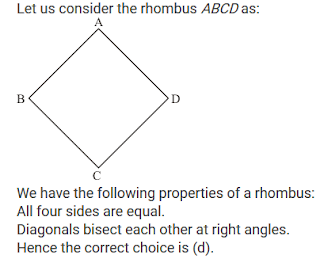5. Diagonals necessarily bisect opposite angles in a
(a) rectangle
(b) parallelogram
(c) isosceles trapezium
(d) square

Solution6. The two diagonals are equal in a
(a) parallelogram
(b) rhombus
(c) rectangle
(d) trapezium

Solution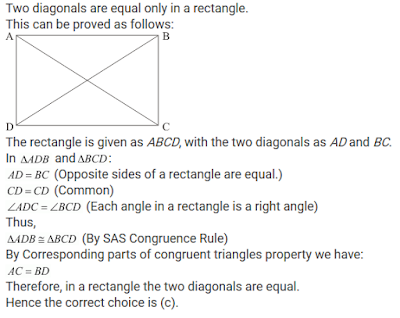7. We get a rhombus by joining the mid-points of the sides of a
(a) parallelogram
(b) rhombus
(c) rectangle
(d) triangle

Solution8. The bisectors of any two adjacent angles of a parallelogram intersect at
(a) 30°
(b) 45°
(c) 60°
(d) 90°

Solution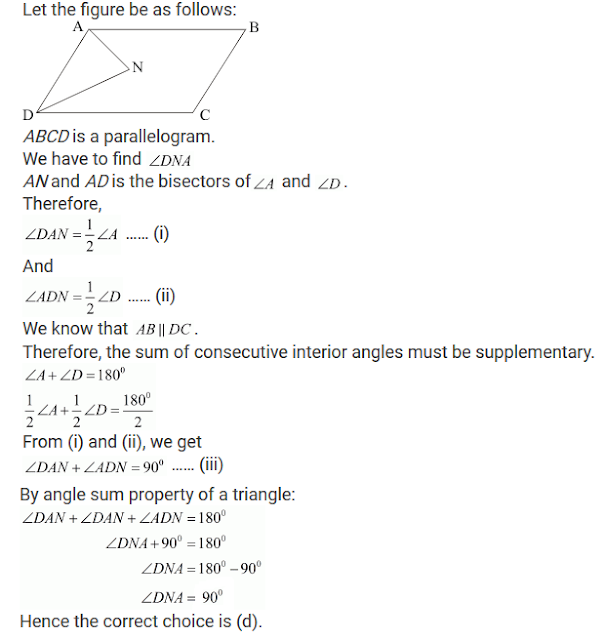9. The bisectors of the angle of a parallelogram enclose a
(a) parallelogram
(b) rhombus
(c) rectangle
(d) square

Solution10. The figure formed by joining the mid-points of the adjacent sides of a quadrilateral is a
(a) parallelogram
(b) rectangle
(c) square
(d) rhombus

Solution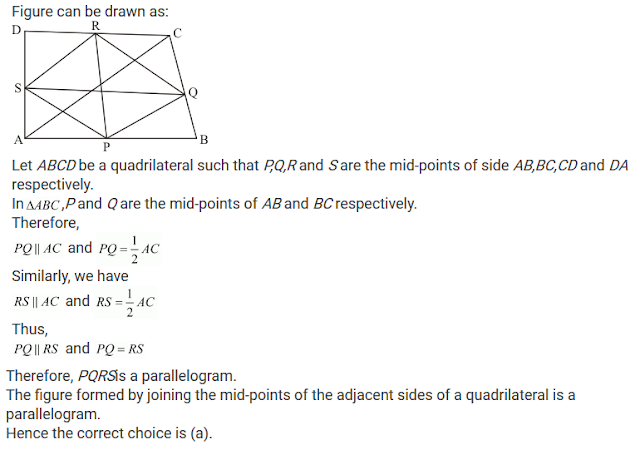11. The figure formed by joining the mid-points of the adjacent sides of a rectangle is a
(a) square
(b) rhombus
(c) trapezium
(d) none of these

Solution12. The figure formed by joining the mid-points of the adjacent sides of a rhombus is a
(a) square
(b) rectangle
(c) trapezium
(d) none of these

Solution13.The figure formed by joining the mid-points of the adjacent sides of a square is a
(a) rhombus
(b) square
(c) rectangle
(d) parallelogram

Solution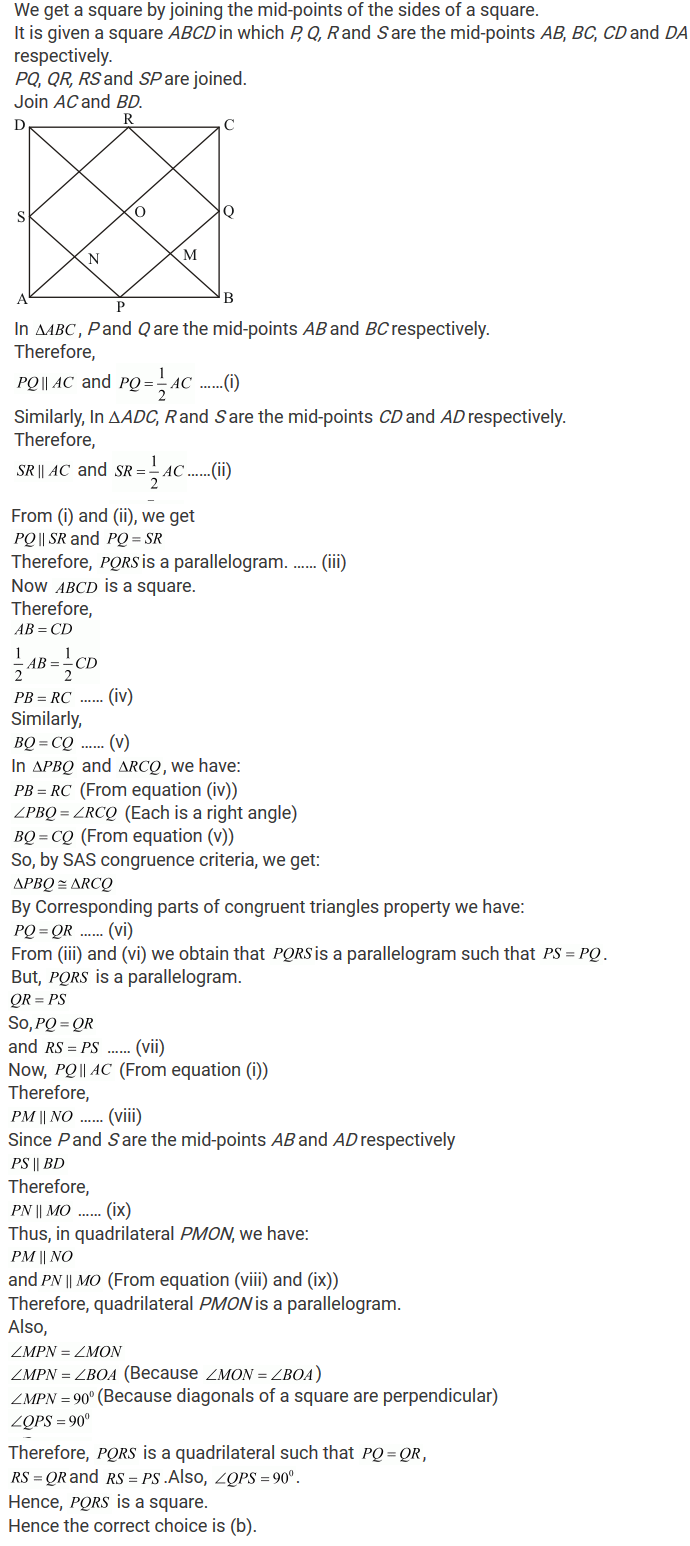14. The figure formed by joining the mid-points of the adjacent sides of a parallelogram is a
(a) rectangle
(b) parallelogram
(c) rhombus
(d) square

Solution15. If one angle of a parallelogram is 24° less than twice the smallest angle, then the measure of the largest angle of the parallelogram is
(a) 176°
(b) 68°
(c) 112°
(d) 102°

Solution16. In a parallelogram ABCD, if ∠DAB = 75° and ∠DBC = 60°, then ∠BDC =
(a) 75°
(b) 60°
(c) 45°
(d) 55°

Solution17. ABCD is a parallelogram and E and F are the centroids of triangles ABD and BCD respectively, then EF =
(a) AE
(b) BE
(c) CE
(d) DE

Solution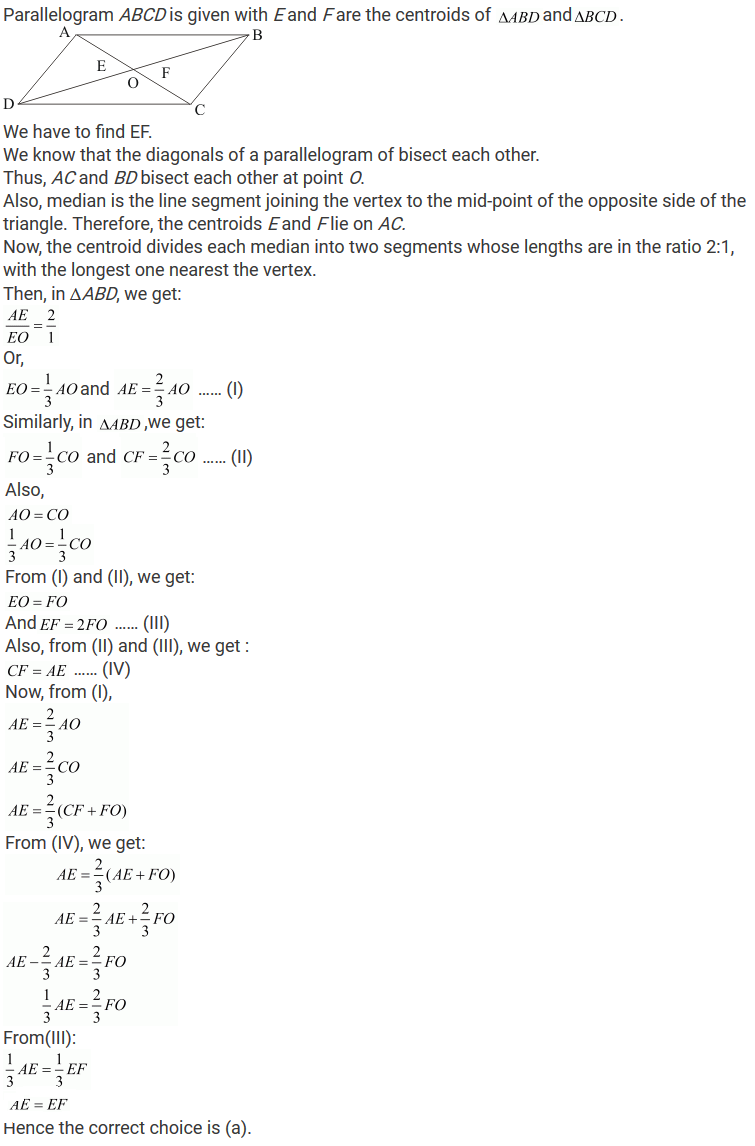18. ABCD is a parallelogram, M is the mid-point of BD and BM bisects ∠B. Then ∠AMB =
(a) 45°
(b) 60°
(c) 90°
(d) 75°

Solution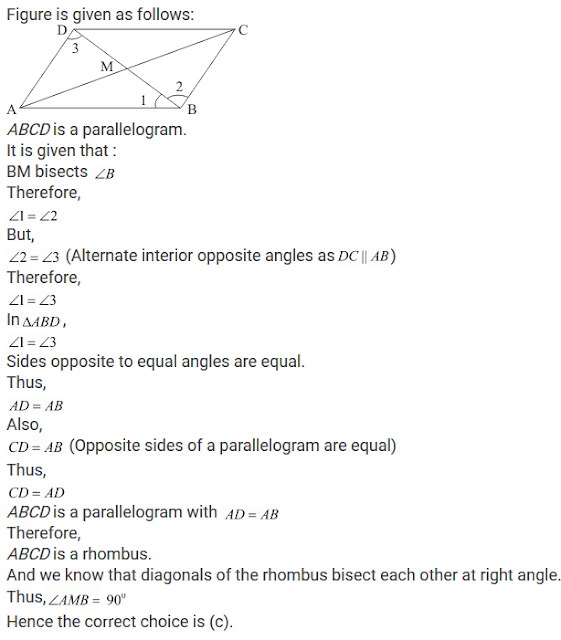19. ABCD is a parallelogram and E is the mid point of BC. DE and AB when produced meet at F. Then AF
(a) 3/2 AB
(b) 2 AB
(c) 3 AB
(d) 5/4 AB

Solution20. If an angle of a parallelogram is two-third of its adjacent angle, the smallest angle of the parallelogram is
(a) 108°
(b) 54°
(c) 72°
(d) 81°

Solution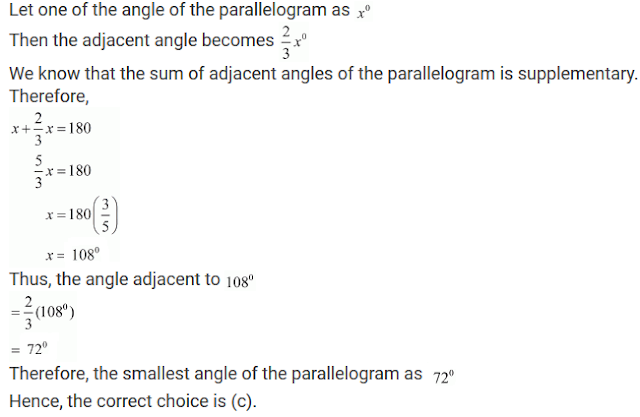21. If the degree measures of the angles of quadrilateral are 4x, 7x, 9x and 10x, what is the sum of the measures of the smallest angle and largest angle?
(a) 140°
(b) 150°
(c) 168°
(d) 180°

Solution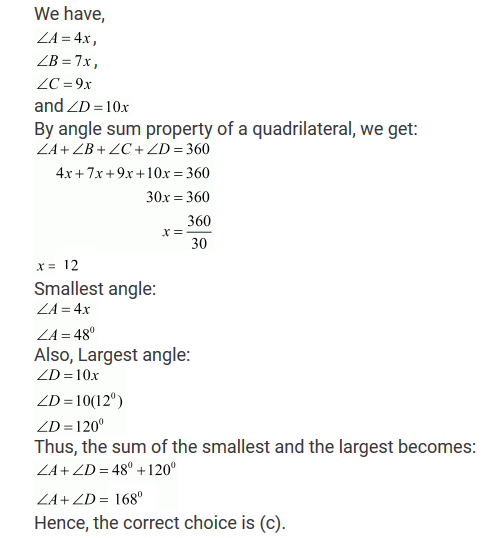22. In a quadrilateral ABCD, ∠A + ∠C is 2 times ∠B + ∠D. If ∠A = 140° and ∠D = 60°, then ∠B =
(a) 60°
(b) 80°
(c) 120°
(d) None of these

Solution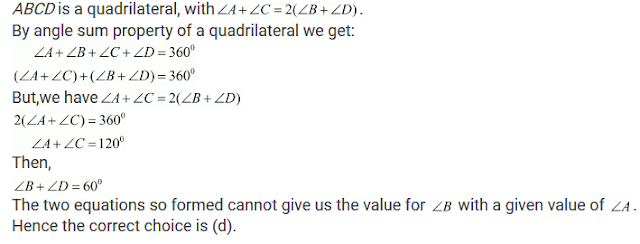23. If the diagonals of a rhombus are 18 cm and 24 cm respectively, then its side is equal to
(a) 16 cm
(b) 15 cm
(c) 20 cm
(d) 17 cm

Solution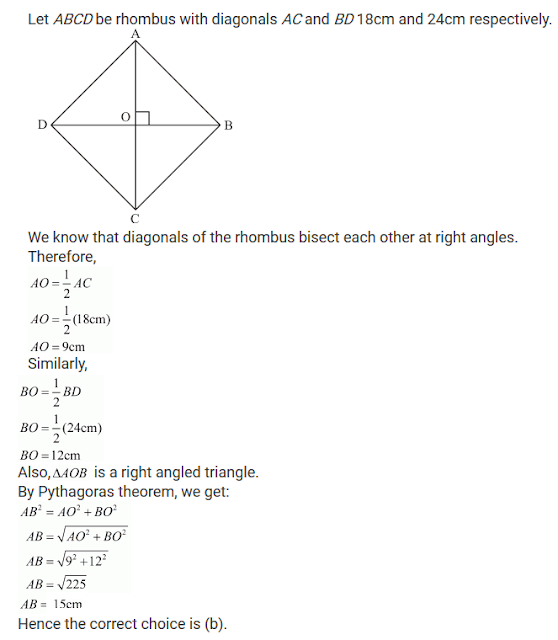24. The diagonals AC and BD of a rectangle ABCD intersect each other at P. If ∠ABD = 50°, then ∠DPC =
(a) 70°
(b) 90°
(c) 80°
(d) 100°

Solution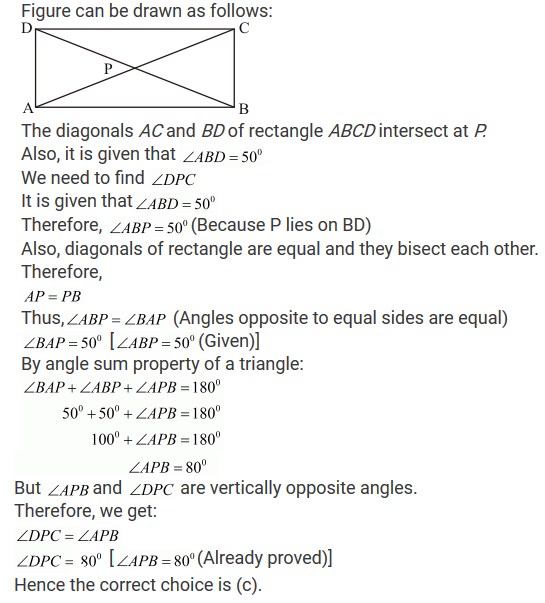25. ABCD is a parallelogram in which diagonal AC bisects ∠BAD. If ∠BAC = 35°, then ∠ABC =
(a) 70°
(b) 110°
(c) 90°
(d) 120°

Solution26. In a rhombus ABCD, if ∠ACB = 40°, then ∠ADB =
(a) 70°
(b) 45°
(c) 50°
(d) 60°

Solution27. In ΔABC, ∠A = 30°, ∠B = 40° and ∠C = 110°. The angles of the triangle formed by joining the mid-points of the sides of this triangle are
(a) 70°, 70°, 40°
(b) 60°, 40°, 80°
(c) 30°, 40°, 110°
(d) 60°, 70°, 50°

Solution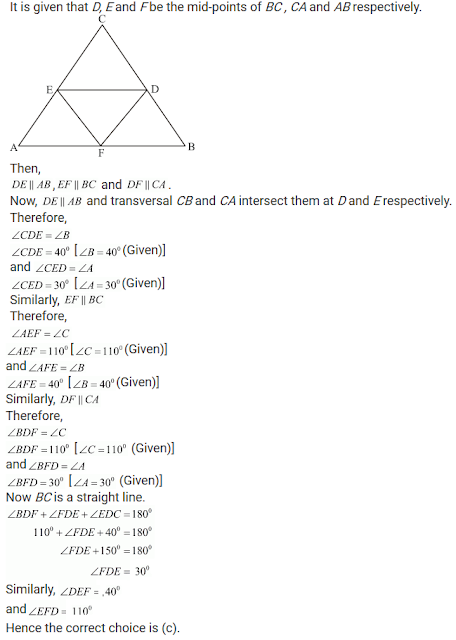28.The diagonals of a parallelogram ABCD intersect at O. If ∠BOC = 90° and ∠BDC = 50°, then ∠OAB =
(a) 40°
(b) 50°
(c) 10°
(d) 90°

Solution29. ABCD is a trapezium in which AB || DC. M and N are the mid-points of AD and the respectively. If AB = 12 cm, MN = 14 cm, then CD =
(a) 10 cm
(b) 12 cm
(c) 14 cm
(d) 16 cm

Solution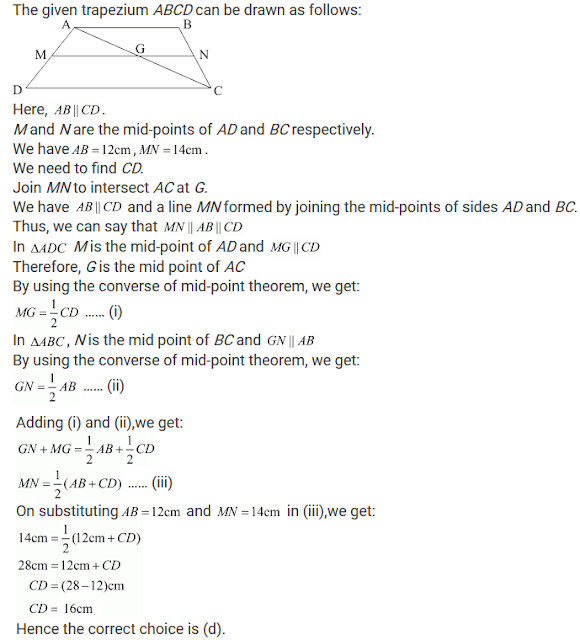30. Diagonals of a quadrilateral ABCD bisect each other. If ∠A= 45°, then ∠B =
(i) 115°
(ii) 120°
(iii) 125°
(iv) 135°

Solution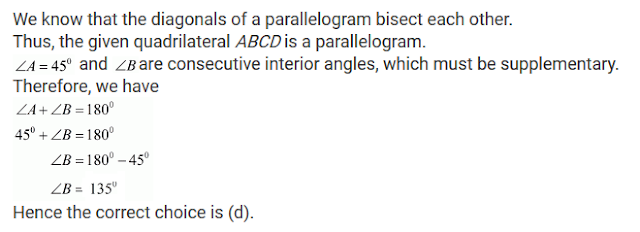31. P is the mid-point of side BC of a parallelogram ABCD such that ∠BAP = ∠DAP. If AD = 10 cm, then CD =
(a) 5 cm
(b) 6 cm
(c) 8 cm
(d) 10 cm

Solution32. In ΔABC, E is the mid-point of median AD such that BE produced meets AC at F. IF AC = 10.5 cm, then AF =
(a) 3 cm
(b) 3.5 cm
(c) 2.5 cm
(d) 5 cm

Solution